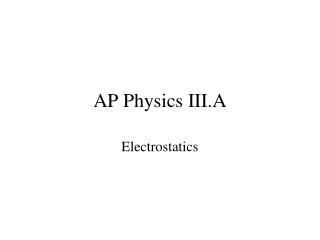DownloadDownload PresentationAP Physics III.A

# AP Physics III.A

Download Presentation## AP Physics III.A

- - - - - - - - - - - - - - - - - - - - - - - - - - - E N D - - - - - - - - - - - - - - - - - - - - - - - - - - -
##### Presentation Transcript

1. AP Physics III.A Electrostatics

2. 18.1 Origin of Electricity

3. 18.2 Charged Objects and Electric Force

4. Law of Conservation of Charge – during any one process, net electrical charge of an isolated system remains constant.

5. Ex. Two identical isolated conducting spheres, one with charge -6 μC and another with net charge +2 μC are allowed to touch. If the two spheres have the same net charge after touching, what is the net charge on each sphere?

6. Attractions and repulsions

7. 18.3 Conductors and Insulators

8. 18.4 Charging by Induction and Conduction (also known as, “I wish I had a decent electroscope”)

9. Charging by Conduction

10. Charging by Induction

11. Induced charge on an insulator

12. 18.5 (Charles De) Coulomb’s Law

13. “Hmm, this looks like something I’ve seen before”

14. Ex. An electron “orbits” the proton of a hydrogen atom at an average distance of 0.53 EE 10-10 m. What is the force that the proton exerts on the electron? What is the velocity of the electron for a circular orbit?

15. Ex. Two charges exert electrical force F on each other. If the magnitude of each charge is doubled and the distance between them is halved, what is the force F′ on each charge in terms of F?

16. Electric forces and vectors

17. Ex. Three Charges in a Line

18. Ex. Three Charges in a Plane

19. III.A.2 Electric Fields and Electric Potential

20. A mass in a gravitational field

21. Charges experience an electrostatic force due to the presence of other charges

22. An electric field is a vector that has a direction that the force exerts on a positive test charge.

23. Some examples

24. Ex. Find the electric force on a proton placed in an electric field of 2.0 EE 4 N/C that is directed along the positive x-axis.

25. Electric fields are vectors. The net electric field at a point in space can be determined by considering the contributions of each charged object and adding them together as vectors.

26. Ex. Electric Field Between Two Point Charges. Two point charges are separated by a distance of 0.100 m. One has a charge of –25.0 μC and the other 50.0 μC. a) What is the magnitude and direction of the electric field at point P between them 0.020 m from the negative charge? b) If an electron is placed at rest at P, what is the magnitude and direction of its initial acceleration?

27. Symmetry and the electric field.

28. Electric Field Lines

29. Notes about field lines • Electric field lines originate on positive charges and terminate on negative charges • The density of the field lines per unit area shows the strength of the field (uniform and non-uniform fields) • Electric field lines are perpendicular to the surface of a charged object • The direction of the field is tangent to any point on the field line • Electric field lines do not cross (Why not?)

30. Field lines around positive and negative charges

31. Field lines between plates of a capacitor.

32. Field lines between two dipoles

33. Field lines between two identical charges

34. Electric Potential Energy

35. Work done on a charge in a uniform electric field

36. Muy importante – the displacement of the charge is in the direction of the electric field.

37. Let’s clarify but not overemphasize the signs

38. Electric Potential Difference

39. Let’s look at “gravitational potential” first

40. So change in electric potential is . . .

41. Electric potential decreases or increases not because the field exerts any more or less force (the field is uniform – like gravity near the Earth’s surface). V changes because of distance. A charge released in the field, traveling a greater distance converts more of its Ue to K (like dropping an object from a greater height).

42. Everyday examples

43. Potential (and therefore potential difference) is scalar (this will simplify some things).

44. Summary • Electric potential energy – energy a charge has because of its potential in an electric field (so far the field is uniform) • Electric potential – electric potential energy per unit charge • Potential difference – change in electric potential